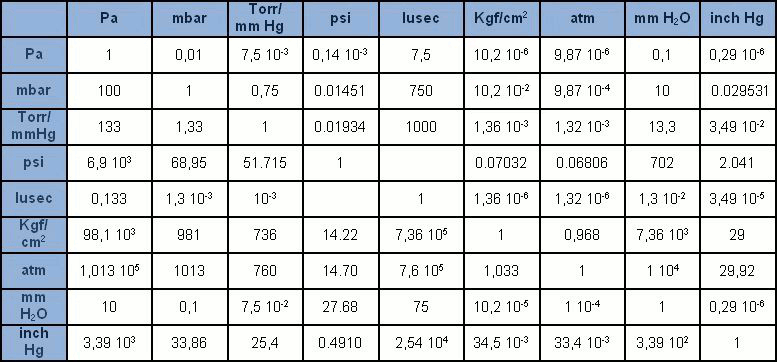PROPERTIES AND LAWS OF GASES provide us with an account of the relations between the state variables, pressure (p), temperature (T) and volume (V), of a given quantity of gas (mass m, number of particles N, molar amount n). The laws are simple relations found experimentally, but which refer to ‘ideal gases’. Gas molecules are considered point-like. Between them there must be no interaction of forces. They collide the one with the other, and with the walls enclosing the space, elastically.

Conceptually, VACUUMS consist in the total absence of matter. While this concept is probably mainly of philosophical significance (Horror Vacui), technically, VACUUMS consist in spaces in which pressures are lower than atmospheric. ABSOLUTE VACUUMS are considered the absence of matter in the space of a volume. However, absolute vacuums cannot be attained. Because gases cannot all be fully removed (hydrogen, oxygen, nitrogen, neon and argon are examples of permanent gases), it is better to define the vacuum as the best vacuum that can be generated in a space. Furthermore, one frequently hears it said that the vacuum is a physical entity, this entity then being opposed to the concept of pressure. Actually, pressure is the only entity used for measurement purposes, for values both higher and lower than atmospheric.

PRESSURE is defined as the result of a force exerted perpendicularly on a unit of surface area. Gases are made up of many constantly moving particles. When these particles collide with a surface, the collisions generate thrust, which is measurable as a force. Pressure is the sum of all the forces produced by the particles and exerted on the unit of surface area. Particles making up gases, when in a state of thermodynamic equilibrium, distribute uniformly in space, hence the composition of the gas is uniform at all points within the container.

The International System (SI) unit of measurement is the pascal [Pa], accounting for the pressure resulting from the force of a newton [N] exerted on a surface area of one square metre [m2]. The pascal is in any case rather a small unit, compared to the units we were once familiar with. It is thus generally given in multiples: hPa = 100Pa (1 mbar), kPa = 1,000Pa (0.1 bar), MPa = 1 million Pa (10 bar). While use of the bar and mbar is accepted, the Pa and its multiples are gradually replacing the many other units of measurement which were once widespread (mmHg, mmH2O, Torr, atm, psi). In the Table, we find all the recurring units of measurement of pressure with their corresponding conversion factors.Atmospheric pressure is approx. 1 absolute bar (1013 mbar). A pressure gauge which indicates zero at atmospheric pressure, and which provides a negative signal in vacuum conditions, measures relative pressure as deviance from atmospheric pressure. Actually, there is no such thing as a negative pressure. In certain cases, a percentage value is used to indicate the value of a vacuum produced, with 100% as absolute vacuum. It is not sufficiently representative, and extension of the field of application renders this indication subjective. It is more correct to express value of pressure, or vacuum, by indicating its absolute value. Since zero-value pressure is in practice unattainable, the indication of the vacuum value shall be a decimal value with many zeroes following the point in accordance with how low the pressure value is, or in other words, how ‘high’ the vacuum value is. For practical purposes logarithmic expressions are used, rather than an endless string of zeroes before a significant number appears. To avoid subjective interpretations, the field of pressures lower than atmospheric, called vacuum, is subdivided with various level indications.By using this site, you agree that we and our partners may set cookies to personalize content according to our Cookies Policy. If you want to know more, click on "More informations" if you do not agree please close this browser window.